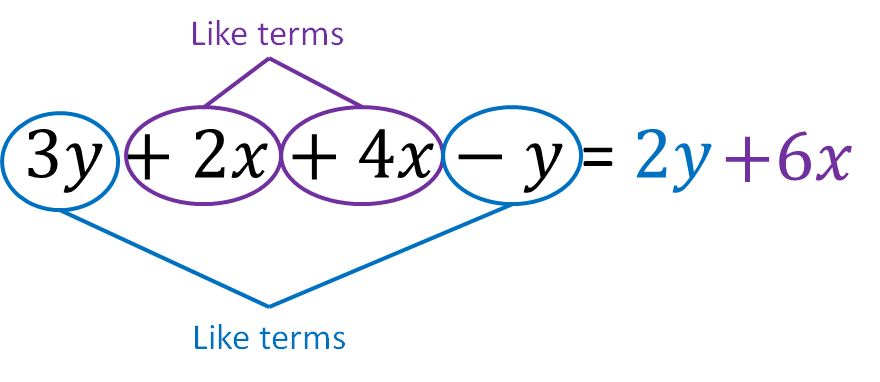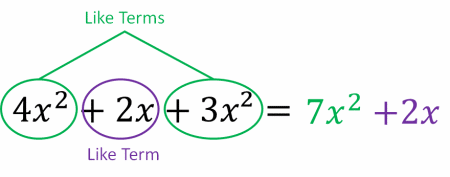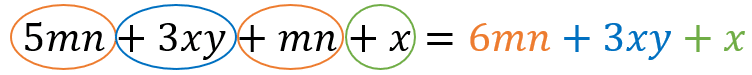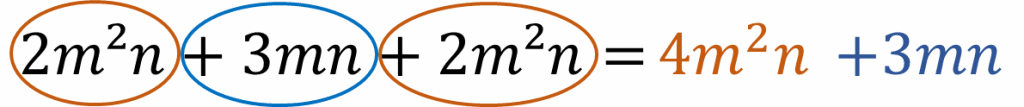# Collecting Like Terms

GCSEKS3Level 1-3AQACambridge iGCSEEdexcelEdexcel iGCSEOCRWJEC

## Collecting Like Terms

A term is an individual part of an expression and typically appears in one of three forms:

• A number by itself
• A letter by itself
• A combination of letters and numbers

Like terms have the same combination of letters. To add or subtract terms with the same letter, we add or subtract the numbers like usual and just put the letter back on the end.

Level 1-3GCSEKS3AQAEdexcelOCRWJECCambridge iGCSEEdexcel iGCSE

## Skill 1: Linear Expressions with a Single Variable

Linear expressions are those in which all variables (i.e. the letters) are to the power of $1$ so there are no squared or cubed terms.

Example: simplify the following,

$2a + 3 + a + 5$

We have to group the terms that are similar; all the terms that are just numbers need grouping together and simplifying, as do the similar algebraic terms.

This gives,

$2a+1a = 3a$

$3 + 5 = 8$

This gives the final answer to be,

$2a + 3 + a + 5 = 3a + 8$

Note: Normally the $1$ in front of the $a$ is left out but we have included it here to make it clear the steps being taken.

Level 1-3GCSEKS3AQAEdexcelOCRWJECCambridge iGCSEEdexcel iGCSE
Level 1-3GCSEKS3AQAEdexcelOCRWJECCambridge iGCSEEdexcel iGCSE

## Skill 2: Like Terms With Different Letters

Sometimes terms have more than one variable (letter) multiplied or divided together:

$\textcolor{red}{xy} + \textcolor{blue}{y} + \textcolor{red}{2xy}$

in this instance, $\textcolor{red}{xy}$ and $\textcolor{red}{2xy}$, are like terms that can be added together to simplify the expression, hence we find,

$\textcolor{red}{3xy} + \textcolor{blue}{y}$

Level 1-3GCSEKS3AQAEdexcelOCRWJECCambridge iGCSEEdexcel iGCSE

## Skill 3: Identifying Like Terms with Powers

Terms of certain powers have to be grouped with terms of the same power. Such terms can also include multiple letters and powers.

$2\textcolor{red}{x^2y} + \textcolor{blue}{xy^2} + 3\textcolor{red}{x^2y} + 5\textcolor{blue}{xy^2} = 5\textcolor{red}{x^2y} + 6\textcolor{blue}{xy^2}$

• $2\textcolor{red}{x^2y}$ and $3\textcolor{red}{x^2y}$ are like terms
• $\textcolor{blue}{xy^2}$ and  $5\textcolor{blue}{xy^2}$ are like terms
Level 1-3GCSEKS3AQAEdexcelOCRWJECCambridge iGCSEEdexcel iGCSE
Level 1-3GCSEKS3AQAEdexcelOCRWJECCambridge iGCSEEdexcel iGCSE

## Example 1: Two Variables

Simplify the expression $3y+2x+4x-y$.

[1 mark]

$\textcolor{blue}{3y}$ and $-\textcolor{blue}{y}$ are like terms

$\textcolor{purple}{2x}$ and $\textcolor{purple}{4x}$ are like termsLevel 1-3GCSEKS3AQAEdexcelOCRWJECCambridge iGCSEEdexcel iGCSE

## Example 2: Including Powers

Simplify the expression $4x^2 +2x +3x^2$.

[1 mark]

$\textcolor{limegreen}{4x^2}$ and $\textcolor{limegreen}{3x^2}$ are like terms

$\textcolor{purple}{2x}$ has no like termsLevel 1-3GCSEKS3AQAEdexcelOCRWJECCambridge iGCSEEdexcel iGCSE

## Example 3: Multiple LettersSimplify the expression $5mn +3xy+mn+x$.

[1 mark]

$\textcolor{maroon}{5mn}$ and $\textcolor{maroon}{mn}$ are like terms

$\textcolor{blue}{3xy}$ and $\textcolor{limegreen}{x}$ have no other like terms

Level 1-3GCSEKS3AQAEdexcelOCRWJECCambridge iGCSEEdexcel iGCSE

## Example 4: Multiple Letters and PowersSimplify the expression $2m^2n+3mn+2m^2n$.

[1 mark]

$\textcolor{maroon}{2m^2n}$ and $\textcolor{maroon}{2m^2n}$ are like terms

$\textcolor{blue}{3mn}$ has no like terms

Level 1-3GCSEKS3AQAEdexcelOCRWJECCambridge iGCSEEdexcel iGCSE

## Collecting Like Terms Example Questions

It is often easier to first group the similar terms together, before simplifying,

$5x+5-2x+3-4-x \\ =(5x-2x-x)+(5+3-4) \\ =2x+4$Gold Standard Education

It is often easier to first group the similar terms together, before simplifying,

$$ab+bc+2ab-bc+a \\ = a+(ab+2ab)+(bc-bc) \\ = a+3ab$$Gold Standard Education

It is often easier to first group the similar terms together, before simplifying:

$$11x+7y-2x-13y \\ = (11x-2x)+(7y-13y) \\ =9x-6y$$Gold Standard Education

It is often easier to first group the similar terms together, before simplifying:

$$2m+6n-3+8n+5n \\ = (2m+5m)+(6n+8n)-3 \\ = 7m+14n-3$$Gold Standard Education

It is often easier to first group the similar terms together, before simplifying:

$$2a^2 + 5b-2a -3b+5a^2\\ = (2a^2+5a^2)+(5b-3b)-2a \\ =7a^2+2b-2a$$